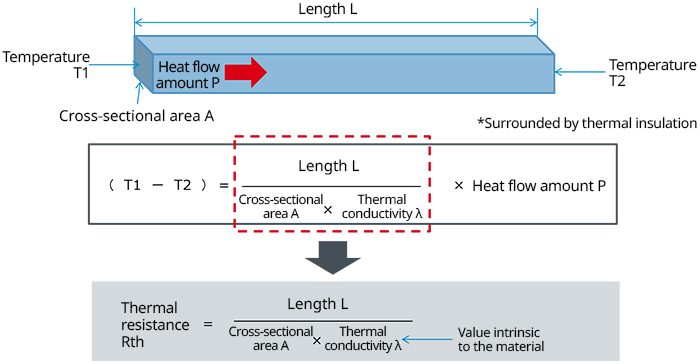# Fundamentals of Thermal Resistance and Heat Dissipation : Thermal Resistance in Conduction

2021.04.21

・In thermal conduction, thermal resistance can be thought of as similar to the electrical sheet resistance of an electrical conductor.

In the previous article, it was explained that heat transmission occurs in three modes, which are conduction, convection, and emission (radiation). From this point, thermal resistance in each of these heat transmission modes is explained. We begin with thermal resistance in conduction.

## Thermal Resistance in Conduction

Conduction of heat is movement of heat between substances or molecules. Thermal resistance in heat condition is represented in the diagram and by the equation below.The diagram illustrates how heat at an end at temperature T1 of an object of cross-sectional area A and length L reaches an end at temperature T2 by conduction.

The first equation indicates that the temperature difference between T1 and T2 is equal to the term surrounded by the red dashed line, multiplied by the heat flow amount P.

The last equation indicates that the term surrounded by the red dashed line is equivalent to the thermal resistance Rth.

The reader has probably grasped this directly from the diagram and the terms in the equation, but in heat conduction, the thermal resistance can basically be thought of as analogous to the electrical sheet resistance of an electrical conductor. It is well known that the sheet resistance is determined using an equation in which resistivity is substituted for the thermal conductivity inside the red dashed line. Just as the resistivity is a value intrinsic to the material of an electrical conductor, the thermal conductivity is also a value intrinsic to the material.

From the equation for the thermal resistance, when the cross-sectional area of the object is increased or the length is shortened, the thermal resistance of the conductor decreases.

The equation to determine （T1－T2） consequently becomes the thermal resistance Rth times the heat flow P, in keeping with the “thermal Ohm’s law” explained in About Thermal Resistance.